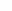COSC 1415 Practice Test #3

1.Declare an array to store 20 numbers that contain a decimal point.

2.Declare an array to store the months of the year as text (e.g. January).

3.Declare an array to store five integers and initialize it with the numbers 1,2,3,8,4

What will the following statements output?  Note: if any of the string operations change the value of a variable, assume all subsequent questions use the new values.

string A = "question";
string B = "I have a ";

4.cout << B + A;

5.cout << B + A.substr(0,3);

6.cout << B.size() - A.size();

7.cout << B.insert(2,"don't") + A;

8.string B = "I have a ";
cout << B.find("a",4);

9.string Car = "Ferrari";
Car.insert(0,"Yellow");

10.cout << Car.substr(0,4) + " " + Car.substr(3,2)+"ud";

11.What library do you have to include to read data from a file?

 for (int X=10; X>1; X=X-2); {    fout << X << " "; }

12.What will the preceding code output to the screen?

What would be the function header line for each of the following?

13.A function to calculate the area of a square.

14.A function to calculate the volume of a sphere.

15.A function to convert gallons to liters.

16.A function to display all the vowels contained in a word.

17.A function that returns true if a number is even or false if the number is odd.

You got out of correct. Your Score: %